# An Extension of Gregus Fixed Point Theorem

## Abstract

Let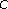be a closed convex subset of a complete metrizable topological vector space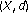and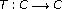a mapping that satisfiesfor all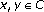, where,,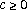,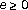,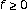, and. Then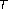has a unique fixed point. The above theorem, which is a generalization and an extension of the results of several authors, is proved in this paper. In addition, we use the Mann iteration to approximate the fixed point of.

## References

1. Greguš M Jr.: A fixed point theorem in Banach space. Bollettino. Unione Matematica Italiana. A. Serie V 1980,17(1):193–198.

2. Murthy PP, Cho YJ, Fisher B: Common fixed points of Greguš type mappings. Glasnik Matematički. Serija III 1995,30(50)(2):335–341.

3. Mukherjee RN, Verma V: A note on a fixed point theorem of Greguš. Mathematica Japonica 1988,33(5):745–749.

4. Olaleru JO: A generalization of Greguš fixed point theorem. Journal of Applied Sciences 2006,6(15):3160–3163.

5. Chidume CE: Geometric properties of Banach spaces and nonlinear iterations. Research Monograph, International Centre for Theoretical Physics, Trieste, Italy, in preparation

6. Kaewcharoen A, Kirk WA: Nonexpansive mappings defined on unbounded domains. Fixed Point Theory and Applications 2006, 2006: 13 pages.

7. Kirk WA: A fixed point theorem for mappings which do not increase distances. The American Mathematical Monthly 1965,72(9):1004–1006. 10.2307/2313345

8. Kannan R: Some results on fixed points. III. Fundamenta Mathematicae 1971,70(2):169–177.

9. Wong CS: On Kannan maps. Proceedings of the American Mathematical Society 1975,47(1):105–111. 10.1090/S0002-9939-1975-0358468-0

10. Chatterjea SK: Fixed-point theorems. Comptes Rendus de l'Académie Bulgare des Sciences 1972, 25: 727–730.

11. Olaleru JO: On weighted spaces without a fundamental sequence of bounded sets. International Journal of Mathematics and Mathematical Sciences 2002,30(8):449–457. 10.1155/S0161171202011857

12. Schaefer HH, Wolff MP: Topological Vector Spaces, Graduate Texts in Mathematics. Volume 3. 2nd edition. Springer, New York, NY, USA; 1999:xii+346.

13. Adasch N, Ernst B, Keim D: Topological Vector Spaces, Lecture Notes in Mathematics. Volume 639. Springer, Berlin; 1978:i+125.

14. Berinde V: On the convergence of the Ishikawa iteration in the class of quasi contractive operators. Acta Mathematica Universitatis Comenianae. New Series 2004,73(1):119–126.

15. Köthe G: Topological Vector Spaces. I, Die Grundlehren der mathematischen Wissenschaften. Volume 159. Springer, New York, NY, USA; 1969:xv+456.

16. Rhoades BE: Comments on two fixed point iteration methods. Journal of Mathematical Analysis and Applications 1976,56(3):741–750. 10.1016/0022-247X(76)90038-X

17. Hardy GE, Rogers TD: A generalization of a fixed point theorem of Reich. Canadian Mathematical Bulletin 1973, 16: 201–206. 10.4153/CMB-1973-036-0

18. Goebel K, Kirk WA, Shimi TN: A fixed point theorem in uniformly convex spaces. Bollettino. Unione Matematica Italiana. Serie IV 1973, 7: 67–75.

19. Olaleru JO: On the convergence of Mann iteration scheme in locally convex spaces. Carpathian Journal of Mathematics 2006,22(1–2):115–120.

## Author information

Authors

### Corresponding author

Correspondence to JO Olaleru.

## Rights and permissions

Reprints and Permissions

Olaleru, J., Akewe, H. An Extension of Gregus Fixed Point Theorem. Fixed Point Theory Appl 2007, 078628 (2007). https://doi.org/10.1155/2007/78628